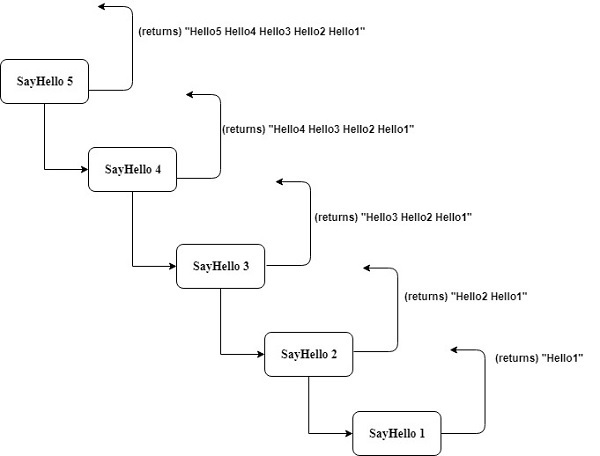# Elm - Loop

Elm is a functional programming language. Elm uses the concept of recursion as an alternative to traditional looping constructs.

This chapter discusses the concept of recursion.

## Recursion

Some computer programming languages allow a module or function to call itself. This technique is known as recursion.

### Illustration

In this program, we will see how to use recursion to display hello five times.

Step 1 − Create a file Loop.elm

Create a module Loop and define a function sayHello. The function sayHello takes an integer value as input and returns a string value.

```module Loop exposing(..)
//function signature
sayHello:Int ->String
//function implementation
sayHello n =
case n of
1 -> "Hello:1 "
_ -> "Hello:" ++ toString (n) ++ " " ++ sayHello(n-1)
```

The function sayHello checks if parameter passed is 1. If the parameter is 1, then function will return, otherwise it will create a string Hello and call the same function.

Step 2 − Invoke sayHello from REPL

Open the elm REPL from current project folder (location of Loop.elm file).

```//import the module Loop
> import Loop exposing(..)
//invoke the sayHello function with parameter value as 5
> sayHello 5
"Hello:5 Hello:4 Hello:3 Hello:2 Hello:1 Hello:0 " : String
>
```### Illustration

The following example prints the sum of n numbers using recursion.

```> sumOfNos n =\
| if n==0 then 0 \
| else (n) + sumOfNos (n-1)
<function> : number -> number1
```

In the elm REPL, we created a function sumOfNos that takes an input number and sums all numbers from 0 to that number.

For example, if we pass input as 5, it will sum up 1+2+3+4+5 which is 15.

```> ssumOfNos 5
15 : number
```

The output of the program is shown above.